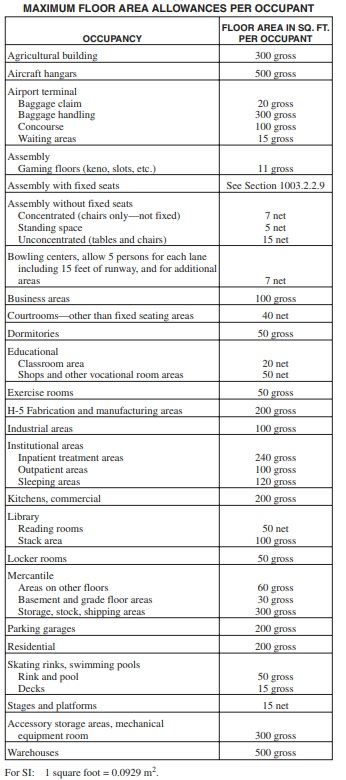# Navigating the Building Code – Part 4 (Occupant Load)

This installment of Navigating the Building Code examines Occupant Load, how to calculate the Occupant Load, and how that calculation can affect the building design.

Occupant Load is defined by the International Building Code as “the number of persons for which the means of egress of a building or portion thereof is designed.” So, what does that actually mean?  In the most general terms, it means it is the maximum amount of people that can occupy a space so that each person can safely exit the space as required.

### How is the Occupant Load Determined?

In order to determine the occupant load, you first must calculate the area of the space in question.  Typically, you must breakdown the project as a whole into smaller parts and determine the area of each individual part.  Each individual part would then be classified by the function of the space.Figure 1.1